# Difference between revisions of "Dual connection"

## Definition

Suppose$E$ is a vector bundle over a differential manifold$M$ and$\nabla$ is a connection on$E$. The dual connection to$\nabla$, denoted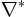$\nabla^*$, is a connection on the dual vector bundle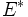$E^*$, defined as follows.

For any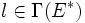$l \in \Gamma(E^*)$ and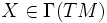$X \in \Gamma(TM)$, we have: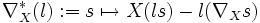$\nabla^*_X(l) := s \mapsto X(ls) - l(\nabla_X s)$

where$s \in \Gamma(E)$

## Motivation

The definition of a dual connection is chosen in such a way that the bilinear form for evaluation: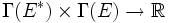$\Gamma(E^*) \times \Gamma(E) \to \R$

satisfies the Leibniz rule. In other wors, we need to ensure that for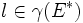$l \in \gamma(E^*)$ and$s \in \Gamma(E)$, we have:$X(ls) = (\nabla^*_X(l))(s) + l(\nabla_X s)$